Maths-
General
Easy

Question

# A and B can do a piece of work in 20 days and B in 15 days. They work together for 2 days and then A goes away. In how many days will B finish the remaining work?Hint:

## The correct answer is: 13 1/2 days.

### Let’s say that the total work can be represented as W.Step 1 of 3:It is given that B can do the whole work(W) in 15 days.So, work done by B in one day =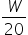……(1)It is given that A and B can do the work in 20 daysSo, work done by A and B in one day =Step 2 of 3:It is given that A and B worked together for 2 days and then A goes away.Work done by A and B in 2 days =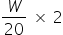=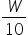Work left for B after A goes away = W - Work done by A and B in 2 days= W -= W -Work left for B after A goes away =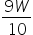Step 3 of 3:Now, we need to find the number of days to completeamount of work.From equation (1) time taken by B to complete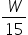work = 1 daytime taken by B to completework =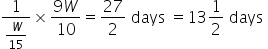Final Answer: Hence, the time taken by B to complete the work after A after 2 days is 13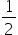days.#### With Turito Foundation.#### Get an Expert Advice From Turito.